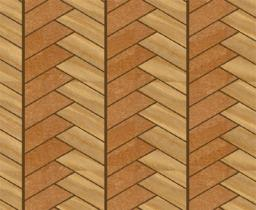# Parquet floor

Three workers laid the parquet floor for 2.301 hour. The first one would do this work alone 7 hours, the second in 6 hours.

For how many hours would fulfill this work the third worker if he worked alone?

x =  8 h

### Step-by-step explanation:Did you find an error or inaccuracy? Feel free to write us. Thank you!

Tips for related online calculators
Looking for calculator of harmonic mean?
Looking for a statistical calculator?
Do you have a linear equation or system of equations and looking for its solution? Or do you have a quadratic equation?
Do you want to convert time units like minutes to seconds?